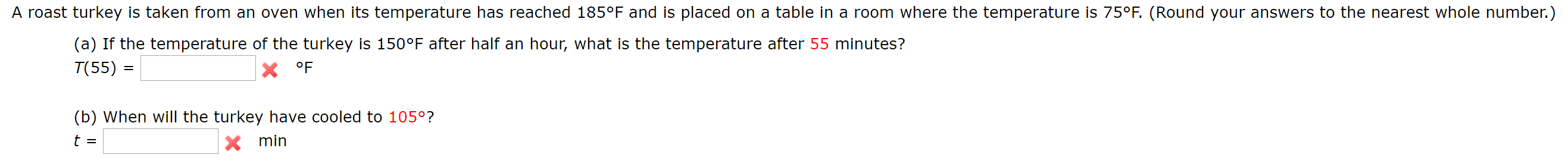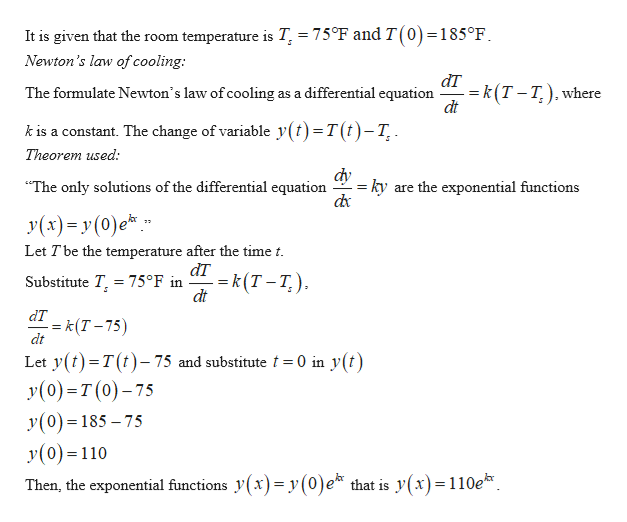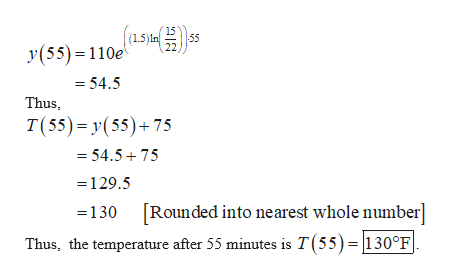# A roast turkey is taken from an oven when its temperature has reached 185°F and is placed on a table in a room where the temperature is 75°F. (Round your answers to the nearest whole number.)(a) If the temperature of the turkey is 150°F after half an hour, what is the temperature after 55 minutes?T(55) =X °F(b) When will the turkey have cooled to 105°?t =Xmin

Question
1246 viewshelp_outlineImage TranscriptioncloseA roast turkey is taken from an oven when its temperature has reached 185°F and is placed on a table in a room where the temperature is 75°F. (Round your answers to the nearest whole number.) (a) If the temperature of the turkey is 150°F after half an hour, what is the temperature after 55 minutes? T(55) = X °F (b) When will the turkey have cooled to 105°? t = Xmin fullscreen
check_circle

Step 1

(a)

Find the temperature after 55 minutes.help_outlineImage TranscriptioncloseIt is given that the room temperature is T = 75°F and T(0) =185°F Newton's law of cooling: dT = k(T-T.), where dt as a differential equation The formulate Newton's law of cooling k is a constant. The change of variable y(t)=T(t)-T, Theorem used: dy = ky The only solutions of the differential equation are the exponential functions У(х) - у (0)e*" Let Tbe the temperature after the time t аT Substitute T, 75°F in k(T-T.) dt dT -= k (T-75) dt Let y () T(t)-75 and substitute 0 in y(t) y(0) T(0)-75 y(0)=185-75 y(0)110 that is y(x)=110e Then, the exponential functions y (x)= y (0)e fullscreen
Step 2

Obtain the value of k as follows.

Step 3

The temperature after 55 minutes...help_outlineImage Transcriptionclose15 -55 (15) n y(55) 110e =54.5 Thus T(55) y(55)+75 54.575 129.5 [Rounded into nearest whole number] -130 Thus, the temperature after 55 minutes is T(55)= |130°F fullscreen

### Want to see the full answer?

See Solution

#### Want to see this answer and more?

Solutions are written by subject experts who are available 24/7. Questions are typically answered within 1 hour.*

See Solution
*Response times may vary by subject and question.
Tagged in
MathCalculus

### Differential Equations